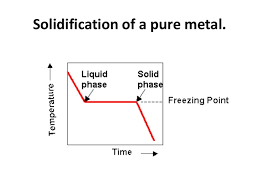## How to Calculate and Solve for Temperature of Solidifying Metals | Solidification of MetalsThe image above represents temperature of solidifying metals.

To compute for temperature of solidifying metals, three essential parameters are needed and these parameters are Surface Temperature (T), Melting Temperature of Metal (Tm) and Initial Temperature of Environment or Mould (To).

The formula for calculating temperature of solidifying metals:

Tsm = T – Tm / To – Tm

Where:

Tsm = Temperature of Solidifying Metals
T = Surface Temperature
Tm = Melting Temperature of Metal
To = Initial Temperature of Environment or Mould

Let’s solve an example;
Find the temperature of solidifying metals when the surface temperature is 12, the melting temperature of metal is 9 and the initial temperature of environment or mould is 10.

This implies that;

T = Surface Temperature = 12
Tm = Melting Temperature of Metal = 9
To = Initial Temperature of Environment or Mould = 10

Tsm = T – Tm / To – Tm
Tsm = 12 – 9 / 10 – 9
Tsm = 3 / 1
Tsm = 3

Therefore, the temperature of solidifying metals is 3 K.

Calculating for Surface Temperature when the Temperature of Solidifying Metals, the Melting Temperature of Metal and the Initial Temperature of Environment or Mould is Given.

T = Tsm (To – Tm) + Tm

Where;

T = Surface Temperature
Tsm = Temperature of Solidifying Metals
Tm = Melting Temperature of Metal
To = Initial Temperature of Environment or Mould

Let’s solve an example;
Find the surface temperature when the temperature of solidifying metals is 10, the melting temperature of metal is 8 and the initial temperature of environment or mould is 14.

This implies that;

Tsm = Temperature of Solidifying Metals = 10
Tm = Melting Temperature of Metal = 8
To = Initial Temperature of Environment or Mould = 14

T = Tsm (To – Tm) + Tm
T = 10 (14 – 8) + 8
T = 10 (6) + 8
T = 68

Therefore, the surface temperature is 68 K.